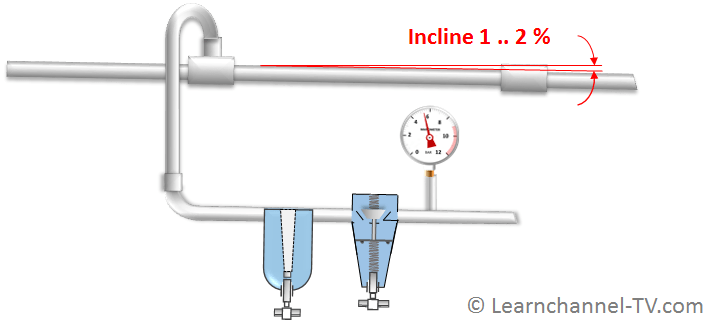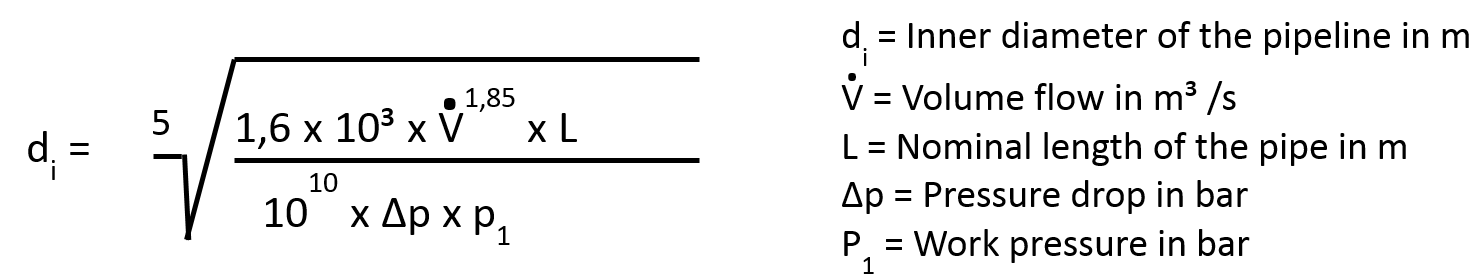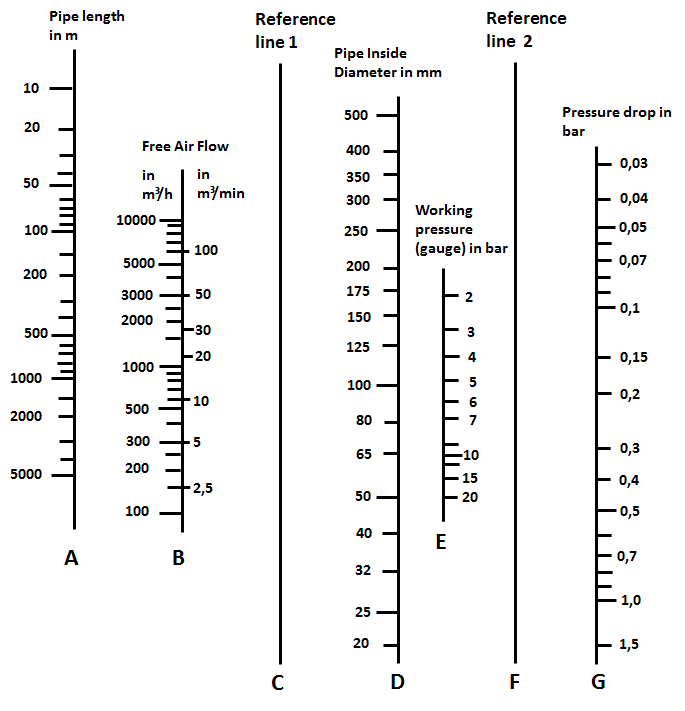### Dimensioning pneumatic lines

Install main pneumatic pipes: To avoid entry of condensation in the pneumatic control , the main pipes are gernerally installed are generally with a gradient of 1 to 2%. At the lowest point, a condensate separator has to be installed. Moreover, branches  to the control parts have to be inserted on the upper side of the main pipes.How to install pneumatic main pipes

How to determine the required pipe inner diameter

The most important criteria for the design of the compressed air supply are:

• The required air volume (volume flow)
• the working pressure (gauge)
• the pipe length
• the maximum pressure drop

Think about that eventually the system will be expanded in the future and more valves and actuators will be connected. The criteria for dimensioning the inside diameter of the pipe are usually:

• arithmetically by means of an approximation formula
• Nomogram (two-dimensional diagram)

Approach 1: approximation formulaApproximation equation for calculating the pipe inner diameter

Approach 2: NomogramPneumatics - Nomogram to determine pipe diameter

How to use this nomogram:

• In line A, enter the pipe length and in line B the necessary volume flow. Connect these 2 points and extend this line so reference line 1 is intersected.
• Likewise, connect the points of line E (working pressure) and line G (maximum pressure drop). This results in an intersection on reference line 2.
• The intersections on the reference lines 1 and 2 are connect to a straight line. This line intersects the line D, where you can now read the required inside diameter.

Work order

You have to plan an installation of a new compressed air supply. What you know: pipe length 200m, volume flow 1000 m³ / h, working pressure above 5 bar and a maximum pressure drop of 0.1 bar. Calculate the required pipe diameter by using the known approximation formula and check this with the help of the nomogram!

In order to take also into account the pressure drop of the pneumatic components as easy as possible, a proper equivalent pipe length is assigned to. Now these equivalent pipe lengths can be added together.pneumatics pressure drop at components

In general: The pressure losses on components and pipes should not exceed 0.3 bar!